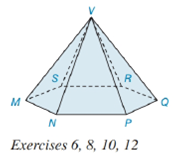Chapter 9.2, Problem 12E### Elementary Geometry for College St...

6th Edition
Daniel C. Alexander + 1 other
ISBN: 9781285195698

#### Solutions

Chapter
Section### Elementary Geometry for College St...

6th Edition
Daniel C. Alexander + 1 other
ISBN: 9781285195698
Textbook Problem
1 views

# Suppose that the base of the hexagonal pyramid in Exercise 6 has an area of 41.6 c m 2 and that the altitude of the pyramid measures 3.7 c m . Find the volume of the hexagonal pyramid.To determine

To find:

The volume of the given pyramid.

Explanation

Given:

The figure given below

and the base of the hexagonal pyramid has an area of 41.6cm2 and the altitude of the pyramid measures 3.7cm.

Formula Used:

The altitude/height of the pyramid is the line segment from the vertex perpendicular to the plane of the base.

The volume V of a pyramid having a base area B and an altitude of length h is given by

V=13Bh.

Calculation:

From the given figure

and the base of the hexagonal pyramid has an area of 41

### Still sussing out bartleby?

Check out a sample textbook solution.

See a sample solution

#### The Solution to Your Study Problems

Bartleby provides explanations to thousands of textbook problems written by our experts, many with advanced degrees!

Get Started